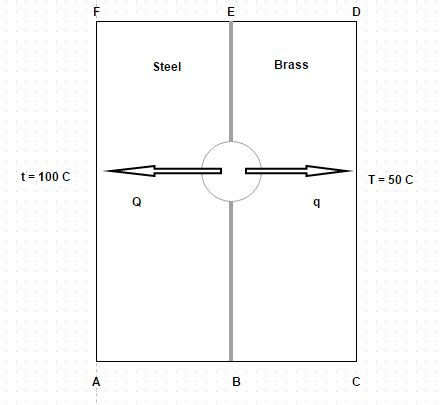# E-PolyLearning

 26. An 8 cm diameter orange, approximately spherical in shape, undergoes ripening process and generates 18000 k J/m³ hr of energy. If external surface of the orange is at 6.5 degree Celsius, find out the temperature at the center of the orange. Take thermal conductivity = 0.8 k J/ m hr degree for the orange material a. 13.5 degree Celsius b. 12.5 degree Celsius c. 11.5 degree Celsius d. 10.5 degree Celsius

 27. A solid sphere of 8 cm radius has a uniform heat generation of 4000000 W/m³. The outside surface is exposed to a fluid at 150 degree Celsius with convective heat transfer coefficient of 750 W/m² K. If thermal conductivity of the solid material is 30 W/m K, determine maximum temperature a. 444.45 degree Celsius b. 434.45 degree Celsius c. 424.45 degree Celsius d. 414.45 degree Celsius
 30. A composite slab consists of 5 cm thick layer of steel (k = 146 kJ/m hr degree) on the left side and a 6 cm thick layer of brass (k = 276 kJ/m hr degree) on the right hand side. The outer surfaces of the steel and brass are maintained at 100 degree Celsius and 50 degree Celsius. The contact between the two slabs is perfect and heat is generated at the rate of 4.2 * 10⁵ k J/m² hr at the plane of contact. The heat thus generated is dissipated from both sides of composite slab for steady state conditions. Calculate the temperature at the interfacea. 115.26 degree celsius b. 125.26 degree celsius c. 135.26 degree celsius d. 145.26 degree celsius